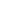# Calculate the simple interest on Rs 4480 at 8%per annum for 3 years?

a) 1035
b) 1075.20
c) 858.50
d) 2330

 1075.20. Solution P = Rs. 4480 R = 8% R = 3 years SI = PNR/100 = (4480 x 8 x 3)/100 = 1075.20
Share :
+
Next
« Prev
Prev
Next »